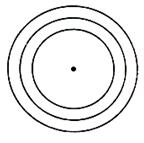#### Thank you for registering.

One of our academic counsellors will contact you within 1 working day.

Click to Chat

1800-5470-145

+91 7353221155

CART 0

• 0
MY CART (5)

Use Coupon: CART20 and get 20% off on all online Study Material

ITEM
DETAILS
MRP
DISCOUNT
FINAL PRICE
Total Price: Rs.

There are no items in this cart.
Continue Shopping

# Please explain me family of circles.The description in given notes is not clear at some points.

one year ago

1. Family of circles having a fixed centreThis equation is given by (x – h)2 + (y – k)2 = r2 (Figure given right)

Since (h, k) is fixed, so the only parameter varying is r. This is one parameter family of circles, and is the equation of the family of concentric circles. Fixation of the radius will give a particular circle.

2. Equation of Family of circles passing through intersection of two circles S= 0 and S2 = 0.

The general equation of the family of circles passing through the intersection of S1 and S2 in given by S1 + kS2 = 0, where k ≠ -1. Here again we have one-parameter (k) equation of family of circles. The particular value of the parameter gives a unique circle.Caution:

If k = –1, we get equation of common chord i.e. straight line instead of circle.

Let S1 ≡ x2 + y2 + 2g1x + 2f1y + c1 = 0

S2 ≡ x2 + y2 + 2g2x + 2f2y + c2 = 0

Since, point lies on both the circles,

⇒ xA2 + yA2 + 2g1xA + 2f1yA + c1 = 0

So, xA2 + yA2 + 2g2xA + 2f2yA + c2 = 0

⇒ xA2 + yA2 + 2g1xA + 2f1yA + c1 + λ (xA2 + yA2  + 2g1xA + 2f1yA + c1) = 0

⇒ Point A(xA, yA) lies on S1 + λ S2 = 0 ∀ λ ∈ R

Similarly point B(xB, yB) lies on S1 + λ S2 = 0 ∀ λ ∈ R

∴ S1 + λ S2 = 0 is the family of circles through the intersection of S1 = 0 and S2 = 0.

3. Equation of a circle circumscribing a triangle whose sides are given by L= 0, L= 0,  L= 0.

This equation is given by L1L2  + λL2L+ µLL= 0, provided coefficeint of xy = 0 and coefficient of x= coefficient of y2.

The particular value of the parameter λ and µ gives a unique circle.

4. Family of circles touching the circle S = 0 and line L = 0 at their point of contact

The required family is given by the equation S + λL = 0, where λ is the required family.